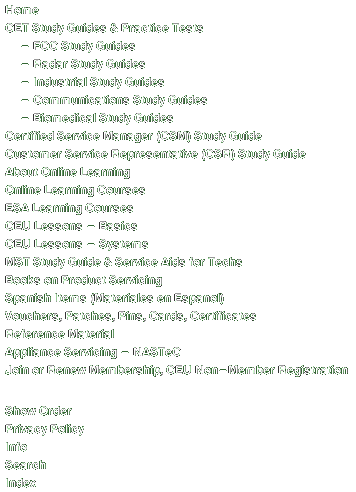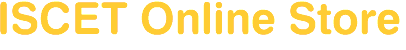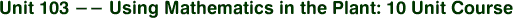Begins by introducing mathematical basics -- numbers, numerals, subtraction, addition, multiplication, etc. Examines common fractions, decimal fractions, ratios, proportions, powers and roots. Discusses the calculator -- usage, basic functions, special functions, internal logic and special purpose calculators. Moves on to cover geometry, algebra, and using formulas for problem solving. Concludes by explaining trigonometry-properties of triangles, trig functions, inverse trig functions and how to use them. Includes the following individual lessons:Lesson 103.1 -- Whole Numbers; Lesson 103.2 -- Common Fractions; Lesson 103.3 -- Decimal Fractions; Lesson 103.4 -- Ratios/Proportions; Lesson 103.5 -- Powers & Roots; Lesson 103.6 -- Calculators; Lesson 103.7 -- Geometry; Lesson 103.8 -- Algebra; Lesson 103.9 -- Using Formulas; Lesson 103.10 -- Trigonometry.069\$89.00